# Soft sphere potential

Jump to: navigation, search

The soft sphere potential is defined as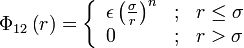$\Phi_{12}\left( r \right) = \left\{ \begin{array}{lll} \epsilon \left( \frac{\sigma}{r}\right) ^n & ; & r \le \sigma \\ 0 & ; & r > \sigma \end{array} \right.$

where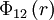$\Phi_{12}\left(r \right)$ is the intermolecular pair potential between two soft spheres separated by a distance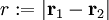$r := |\mathbf{r}_1 - \mathbf{r}_2|$,$\epsilon$ is the interaction strength and$\sigma$ is the diameter of the sphere. Frequently the value of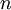$n$ is taken to be 12, thus the model effectively becomes the high temperature limit of the Lennard-Jones model . If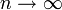$n\rightarrow \infty$ one has the hard sphere model. For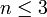$n \le 3$ no thermodynamically stable phases are found.

## Equation of state

The soft-sphere equation of state has recently been studied by Tan, Schultz and Kofke  and expressed in terms of Padé approximants. For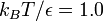$k_BT/\epsilon=1.0$ and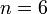$n=6$ one has (Eq. 8):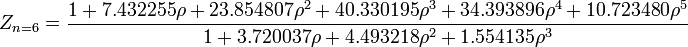$Z_{n=6} = \frac{1 + 7.432255 \rho + 23.854807 \rho^2 + 40.330195 \rho^3 + 34.393896 \rho^4 + 10.723480 \rho^5}{1+ 3.720037 \rho + 4.493218 \rho^2 + 1.554135 \rho^3}$

and for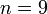$n=9$ one has (Eq. 9):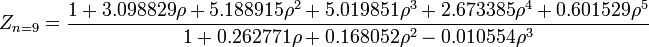$Z_{n=9} = \frac{1 + 3.098829 \rho + 5.188915 \rho^2 + 5.019851 \rho^3 + 2.673385 \rho^4 + 0.601529 \rho^5}{1+ 0.262771 \rho + 0.168052 \rho^2 - 0.010554 \rho^3}$

## Virial coefficients

Tan, Schultz and Kofke have calculated the virial coefficients at$k_BT/\epsilon=1.0$ (Table 1):

 n=12 n=9 n=6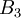$B_3$ 3.79106644 4.27563423 5.55199919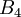$B_4$ 3.52761(6) 3.43029(7) 1.44261(4)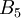$B_5$ 2.1149(2) 1.08341(7) -1.68834(9)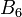$B_6$ 0.7695(2) -0.21449(11) 1.8935(5)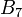$B_7$ 0.0908(5) -0.0895(7) -1.700(3)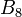$B_8$ -0.074(2) 0.071(4) 0.44(2)

## Melting point

For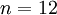$n=12$

 pressure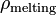$\rho_{\mathrm {melting}}$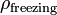$\rho_{\mathrm {freezing}}$ Reference 22.66(1) 1.195(6) 1.152(6) Table 1  23.24(4) 1.2035(6) 1.1602(7) Table 2 

For$n=9$

 pressure$\rho_{\mathrm {melting}}$$\rho_{\mathrm {freezing}}$ Reference 36.36(10) 1.4406(12) 1.4053(14) Table 3 

For$n=6$

 pressure$\rho_{\mathrm {melting}}$$\rho_{\mathrm {freezing}}$ Reference 100.1(3) 2.320(2) 2.295(2) Table 4